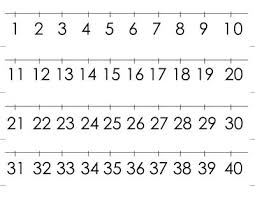# Probability 71204

On ten identical cards, there are numbers from zero to nine. Determine the probability that a two-digit number randomly drawn from the given cards is:
a) even
b) divisible by six
c) divisible by twenty-one

p1 =  0.5062
p2 =  0.1728
p3 =  0.0494

### Step-by-step explanation:Did you find an error or inaccuracy? Feel free to write us. Thank you!

Tips for related online calculators
Need help calculating sum, simplifying, or multiplying fractions? Try our fraction calculator.